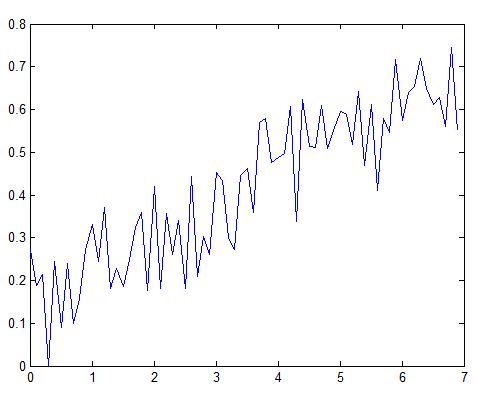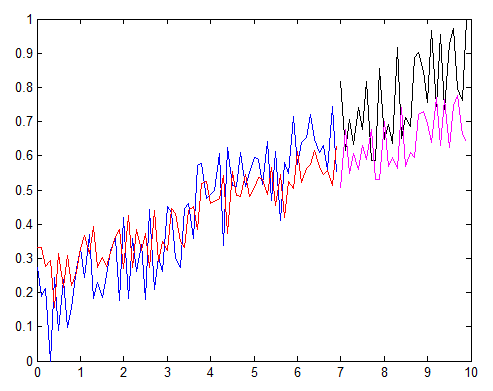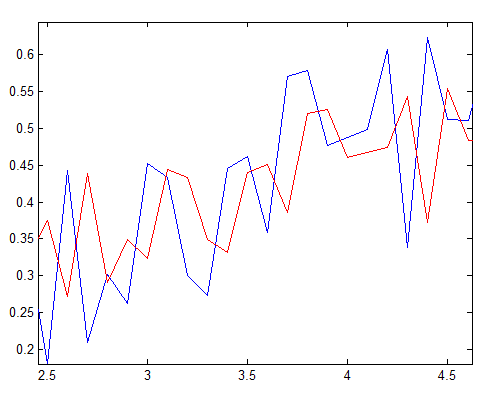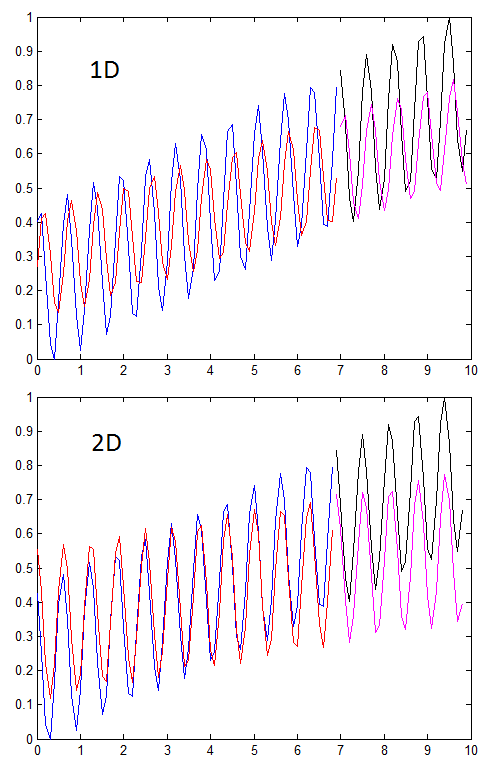# SVM regression on time series, is there a lag?

It would be nice if we can predict the future. For example, give the following time series, can we predict the next point?Let’s use SVM regression, which is said to be powerful. We use the immediate past data point as the predictor. We train our model with the first 70% of data. Blue and Black are actual data, and Red and Pink are predicted data.The prediction in general matches the trend. But if you look closely, you see that the predicted data is always lagging the actual data by one time step. See a zoom in below.Why does this lag come from?

Let’s plot the predictor and the predicted (i.e. the current data point vs the next data point):

It took me a few hours to think about this. Well, the reason turns out to be simple. It’s because our SVM model is too simple (only taking the last data point as predictor): if a data has a increasing trend, then the SVM model, which only consider the immediate history, will give a high predicted value if the current data value is high, a low value if the current data value is low. As a consequence, the predicted value is actually more similar to the current value – and that gives a lag if compared to the actual data.

To reduce the lag, you can build a more powerful SVM model – say use the past 2 data points as the predictor. It will make a more reliable prediction – if the data is not random. See below comparison: you can easily see the lag is much smaller.Don't want to miss new papers in your field? Check out Stork we developed:## 8 Replies to “SVM regression on time series, is there a lag?”

1.Riccardo Perego says:

Hi Mr Cui,
I have found the same situation that you described here in this post.
I tried to add more data points before the day that I want to predict, despite that the lag is still there. How is possible that? I have to change in manual way some weights in the the SMV Function about these previous data in order to obtain a future value?

2.Nabiil Aujeemuddee says:

Hi Xu, I need to run the same on python or R. Do you have the codes please. Thanks.

3.Xu Cui says:

@Nabiil Aujeemuddee
Unfortunately I don’t have python or R code …

4.Lawrence says:

Hi Xu Cui,

1)Which part of your source codes correspond to the lag reduction?
2)What if my data do not have a trend? If it’s random, can the code be used to reduce the lag?

5.Asghar Ali says:

how many values we take lag and why? Using R in Svm.

1.Xu Cui says:

I don’t quite understand your question, Asghar.

6.Asghar Ali says:

why we take lages using Svm in R.
How many lages values we take?

1.Xu Cui says:

I unfortunately have little experience in SVM using R.